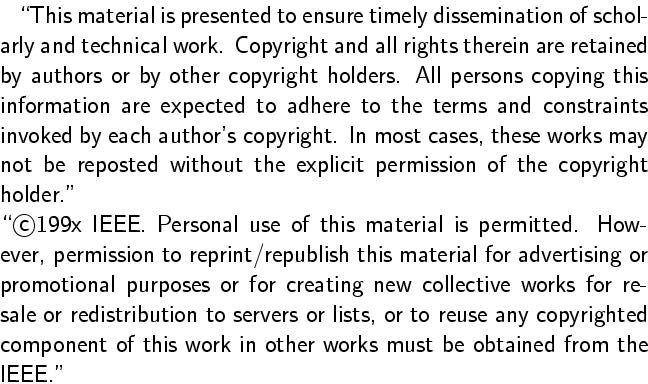Stephanie Geist, Gunther Reißig, and Jörg Raisch.
On the convexity of reachable sets of nonlinear dynamic systems - an important step in generating discrete abstractions of continuous systems.
Proc. 11th IEEE Int. Conf. Methods and Models in Automation and Robotics (MMAR), Miedzyzdroje, Poland, Aug. 29 - Sep. 1, 2005, pp. 101-106, S. Domek and R. Kaszynski, eds., 2005.
Full text. (Free access.)Abstract:
Given a flow $φ:U⊆ T × X → X$ of some ordinary differential equation , subsets $Ω$1, Ω2⊆X of the state space, and some $τ>0$ with $[0,τ]×Ω$1⊆U , we investigate whether $φ(τ,Ω$1) and $Ω$2 intersect. In other words, we examine, whether $Ω$2 is reachable from $Ω$1 after elapsed time $τ$. This problem plays a central part in a recently proposed approach to systems analysis via discrete abstractions, where $Ω$1 and $Ω$2 are members of a covering of the state space of $(∗)$. We propose to view this problem as an optimisation problem, which is convex iff $Ω$1 and $Ω$2 are so. We derive conditions under which the image of a set under some $C∞$ -diffeomorphism is convex and show that these results apply to the time-$τ$ -map $φ(τ,·)$ of $(∗)$ under mild conditions on $f$. We further show that the image of a ball under the time-$τ$ -map is convex provided its radius is sufficiently small and give an upper bound on that radius depending on $τ$ and the first and second derivatives of $f$ only. This shows that the approach proposed in this paper, namely, to treat the reachability problem arising in the context of discrete abstractions as an optimisation problem, applies to virtually any differential equation ($∗$) and yields convex problems provided that the state space is covered by sufficiently small balls.
BibTeX entry:
@InProceedings{GeistReissigRaisch05,
author = {Stephanie Geist and Gunther Rei{\ss}ig and J{\"o}rg Raisch},
title = {On the convexity of reachable sets of nonlinear dynamic systems -- an important step in generating discrete abstractions of continuous systems},
booktitle = {Proc. 11th IEEE Int. Conf. Methods and Models in Automation and Robotics (\nobreak{MMAR}), Miedzyzdroje, Poland, } # aug # { 29-} # sep # { 1, 2005},
year = 2005,
pages = {101-106},
editor = {S. Domek and R. Kaszy{\'n}ski},
isbn = {83-60140-85-5 (abstracts printed), 83-60140-90-1 (full paper on CD)}
}


Impressum und Haftungsausschluß